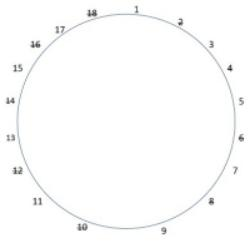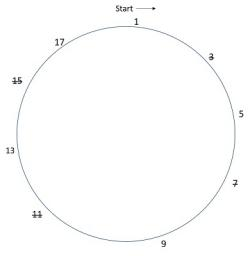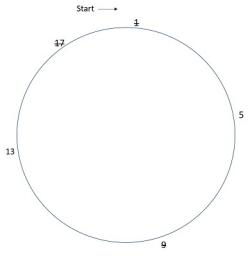# Games and Tournament - Logical Reasoning (MCQ) Questions for Q. 29709

• From a group of N contenders, a party has to select a leader.
• Even after holding a series of meetings, the politicians and the general body failed to reach a consensus.
• It was then proposed that all N contenders be given a number from 1 to N. Then they will be asked to stand on a podium in a circular arrangement, and counting would start from the contender numbered 1.
• The counting would be done in a clockwise fashion.
• The rule is that every alternate contender would be asked to step down as the counting continued, with the circle getting smaller and smaller, till only one person remains standing. Therefore, the first person to be eliminated would be the contender number 2.
If N is 18, which position should a contender choose if he has to be the leader?

- Published on 03 May 17

a. 3
b. 5
c. 11
d. 17

In this question, it would be about identifying a pattern. If you start small, you will find this pattern:

When N is a power of 2, the number of the leader that gets selected is 1 and after that it moves in a sequence of odd numbers till the next power hits.

Once you get the sequence, you would be able to solve the question.

We need to find for N = 18.

Nearest power of 2 is 16, for which the number of leader would be 1.

Every subsequent jump of 1 in N will result in a jump of 2 in number of leader.

Jumps in N = 18 - 16 = 2
Jump in number of Leader = 2 x 2 = 4

So, the number of leader who will get selected is 1 + 4 = 5.After 3 rounds 5 and 13 will remain, beginning with 5, 13 will have to step down.

Thus, 5 will be the leader.

## ➨ Post your comment / Share knowledgeEnter the code shown above:

(Note: If you cannot read the numbers in the above image, reload the page to generate a new one.)